## Laplace Transform

The Laplace transform is an Integral Transform perhaps second only to the Fourier Transform in its utility in solving physical problems. Due to its useful properties, the Laplace transform is particularly useful in solving linear Ordinary Differential Equations such as those arising in the analysis of electronic circuits.

The (one-sided) Laplace transform(not to be confused with the Lie Derivative) is defined by(1)

whereis defined for. A two-sided Laplace transform is sometimes also defined by(2)

The Laplace transform existence theorem states that, ifis piecewise Continuous on every finite interval in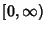satisfying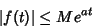(3)

for all, then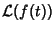exists for all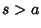. The Laplace transform is also Unique, in the sense that, given two functionsandwith the same transform so that(4)

then Lerch's Theorem guarantees that the integral(5)

vanishes for allfor a Null Function defined by(6)

The Laplace transform is Linear since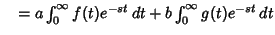(7)
The inverse Laplace transform is given by the Bromwich Integral (see also Duhamel's Convolution Principle). A table of several important Laplace transforms follows.Range 1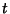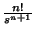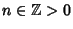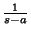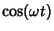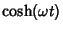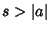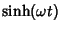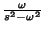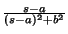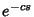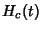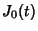In the above table,is the zeroth order Bessel Function of the First Kind,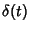is the Delta Function, andis the Heaviside Step Function. The Laplace transform has many important properties.

The Laplace transform of a Convolution is given by(8)(9)

Now consider Differentiation. Letbe continuously differentiable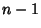times in. If, then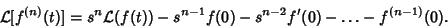(10)

This can be proved by Integration by Parts,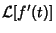(11)

Continuing for higher order derivatives then gives(12)

This property can be used to transform differential equations into algebraic equations, a procedure known as the Heaviside Calculus, which can then be inverse transformed to obtain the solution. For example, applying the Laplace transform to the equation(13)

gives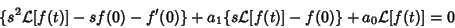(14)(15)

which can be rearranged to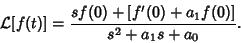(16)

If this equation can be inverse Laplace transformed, then the original differential equation is solved.

Consider Exponentiation. If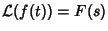for, thenfor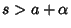.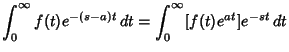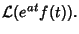(17)

Consider Integration. Ifis piecewise continuous and, then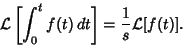(18)

The inverse transform is known as the Bromwich Integral, or sometimes the Fourier-Mellin Integral.

See also Bromwich Integral, Fourier-Mellin Integral, Fourier Transform, Integral Transform, Laplace-Stieltjes Transform, Operational Mathematics

References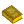Laplace Transforms

Abramowitz, M. and Stegun, C. A. (Eds.). Laplace Transforms.'' Ch. 29 in Handbook of Mathematical Functions with Formulas, Graphs, and Mathematical Tables, 9th printing. New York: Dover, pp. 1019-1030, 1972.

Arfken, G. Mathematical Methods for Physicists, 3rd ed. Orlando, FL: Academic Press, pp. 824-863, 1985.

Churchill, R. V. Operational Mathematics. New York: McGraw-Hill, 1958.

Doetsch, G. Introduction to the Theory and Application of the Laplace Transformation. Berlin: Springer-Verlag, 1974.

Franklin, P. An Introduction to Fourier Methods and the Laplace Transformation. New York: Dover, 1958.

Jaeger, J. C. and Newstead, G. H. An Introduction to the Laplace Transformation with Engineering Applications. London: Methuen, 1949.

Morse, P. M. and Feshbach, H. Methods of Theoretical Physics, Part I. New York: McGraw-Hill, pp. 467-469, 1953.

Spiegel, M. R. Theory and Problems of Laplace Transforms. New York: McGraw-Hill, 1965.

Widder, D. V. The Laplace Transform. Princeton, NJ: Princeton University Press, 1941.